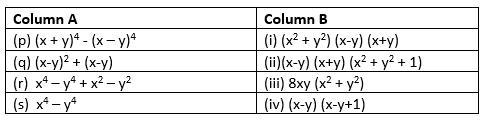# Worksheet for Factorization Class 8

In this page we have Worksheet for Factorization Class 8 Chapter 14 CBSE Maths .This worksheet has all format of questions covering the whole chapter. Questions format is Multiple choice questions, Match the column, Fill in the blank, short answer type. Hope you like them and do not forget to like , social share and comment at the end of the page.

Question 1
Factorize the following expression
(a) x(1 - b) - y(1 - b)
(b) 9r(z + 1) + 3r(z+ 1)
(c) p2 + q2 + 5a(p2 + q2)
(d) x² + x y + 8x + 8y
(e) 3(x + y) - 9(x + y)2
(f) l(3m – 7n) - n(3m – 7n)
(g) (2m – 5) (3a - 2b) - (2m – 5) (2b – 3a)
(h) x(1 + y) + (7 + 7y)
(i) (6xy + 3x) + (2y + 1)
(j) x(y – z)2 – a(z - y)3
(k) z – 7 + 7 x y – x y z

(a) x(1 - b) - y(1 - b) =(1-b)(x-y)
(b) 9r(z + 1) + 3r(z+ 1)=(z+1)(9r+3r)=12r(z+1)
(c) p2+ q2+ 5a(p2+ q2)=1(p2+ q2) + 5a(p2+ q2)=(1+5a)(p2+ q2)
(d) x² + x y + 8x + 8y=x(x+y) + 8(x+y)=(x+8)(x+y)
(e) 3(x + y) - 9(x + y)2=3(x+y)[1-3(x+y)]=3(x+y)(1-3x-3y)
(f) l(3m – 7n) - n(3m – 7n)=(l-n)(3m-7n)
(g) (2m – 5) (3a - 2b) - (2m – 5) (2b – 3a)=(2m-5)[3a-2b -(2b-3a)]=6(2m-5)a
(h) x(1 + y) + (7 + 7y)=(x+7)(1+y)
(i) (6xy + 3x) + (2y + 1)=(3x+1)(2y+1)
(j) x(y – z)2 – a(z - y)3=(y-z)2[x-a(z-y)]=(y-z)2(x-az+ay)
(k) z – 7 + 7 x y – x y z=1( z-7) -xy(z-7)=(1-xy)(z-7)

Question 2
Work out the following divisions.
(i) (11x – 121) ÷ 11
(ii) (15x – 25) ÷ (3x – 5)
(iii) 10y(9y + 21) ÷ 2(3y + 7)
(iv) 9p²q² (3z – 12) ÷ 27pq(z – 4)

(i) (11x – 121) ÷ 11= 11(x-11)÷ 11=(x-11)
(ii) (15x – 25) ÷ (3x – 5)=5(3x-5) ÷ (3x – 5)= 5
(iii) 9p²q² (3z – 12) ÷ 27pq(z – 4)= pq

Question 3
Factorize the following expressions.
(i) z² + 6z + 8
(ii) z² – 10z + 21
(iii) z² + 6z – 16

(i) z² + 6z + 8 = z² + 2z + 4z+8 = z(z+2) + 4(z+2)=(z+4)z+2)
(ii) z² – 10z + 21 = z² -7z -3z+21= z(z-7) -3(z-7)=(z-3)(z-7)
(iii) z² + 6z – 16=z² + 8z -2z- 16=z(z+8)-2(z+8)=(z-2)(z+8)

## True and False

Question 4
True and False
(i) $x^2 + 4x + 3$ is factorised as (x+1)(x+3)
(ii)$x^2 + (a + b)x + ab = (a + b) (x + ab)$
(iii) h is a factor of $2\pi (h + r)$.
(iv) Factors of $(96-4x -x^2)$ is (x+12)(8-x)
(v)Common factor of $17abc$, $34ab^2$, $51a^2b$ is 17ab

(i) True
(ii) false
(iii) False
(iv) True
(v) True

## Fill in the blanks

Question 5
Fill in the blanks
(i) $-x + x^3$ is factorised as ______
(ii)$\frac {x^2 + 5xy-24y^2}{x+ 8y}$ = __________
(iii) Factorised form of (x-10)(x+7) + 16 is _______
(iv) Factorised form of 23xy – 46x + 54y – 108 is _____
(v)Factorized form of $a^{12}x^{4} -a^{4}x^{12}$ is ____________

(i) x(x-1)(x+1)
(ii) (x- 3y)
(iii) (x-9)(x+6)
(iv) (23x + 54) (y – 2)
(v) $a^4x^4(a^4 + x^4)(a^2 + x^2)(a+x)(a-x)$

## Multiple Choice Questions

Question 6
The radius of a circle is 7ab – 7bc – 14a cm, then circumference of the circle is ( Given $\pi =\frac {22}{7}$)
(a) 22(ab -bc-2a)
(b) 44(ab -bc-2a)
(c)(ab -bc-2a)
(d) None of these
Question 7
Factorised form of $x^2 -(p-5)x -5p$ is
(a) (x-5)(x-p)
(b) (x+5)(x+p)
(c)(x-p)(x+5)
(d) (x+p)(x-5)
Question 8
Factorised form of $r^2 – 10r + 21$ is
(a) (r - 7) (r - 3)
(b) (r -7) (r +3)
(c)(r +1) (r - 4)
(d) (r -1) (r - 4)
Question 9
The expression $\frac {6x^2 -17x +12}{3x-4}$ is
(a) (2x+3)
(b) (3x+2)
(c)(2x -3)
(d) (3x-2)

(6) (b)
(7) (c)
(8) (a)
(9) (c)

## Match the column

Question 10(p) => (iii)
(q) => (iv)
(r) => (ii)
(s) => (i)

## Summary

This factorisation class 8 worksheet with answers is prepared keeping in mind the latest syllabus of CBSE . This has been designed in a way to improve the academic performance of the students. If you find mistakes , please do provide the feedback on the mail.